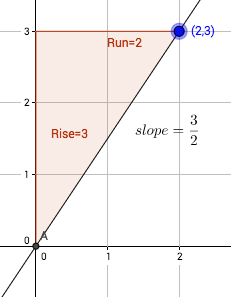IM1.7.2 Slope Triangles 1: Perpendicular

Author:
Lind, Jon
Topic:
Triangles

Slope TrianglesThe image above shows a slope triangle for the line through (0,0) and (2,3). We're going to use slope triangles to make some more connections between algebra and geometry.

Using Slope Triangles: Perpendicular

1. Construct a line from the origin (point O) to the point (a,b). Label this line L. 2. Construct a slope triangle for this line. 3. Rotate your slope triangle and point (a,b) 90° counter-clockwise around point O. 4. Construct a new line based on this slope triangle. Label this line M.

1. What is the slope of line L? 2. What is the slope of line M? 3. What is the relationship between these two lines (use AL)? 4. Would this relationship change if we translated the intersection point (O)? 5. Would this relationship change if we rotated the whole picture? 6. What is the relationship between slopes of perpendicular lines (use AL)? 7. If I had a new line N with a slope of , what would be the slope of a line perpendicular to N?